Courses

# Modelling of Control Systems - 1

## 10 Questions MCQ Test GATE ECE (Electronics) 2022 Mock Test Series | Modelling of Control Systems - 1

Description
This mock test of Modelling of Control Systems - 1 for Electrical Engineering (EE) helps you for every Electrical Engineering (EE) entrance exam. This contains 10 Multiple Choice Questions for Electrical Engineering (EE) Modelling of Control Systems - 1 (mcq) to study with solutions a complete question bank. The solved questions answers in this Modelling of Control Systems - 1 quiz give you a good mix of easy questions and tough questions. Electrical Engineering (EE) students definitely take this Modelling of Control Systems - 1 exercise for a better result in the exam. You can find other Modelling of Control Systems - 1 extra questions, long questions & short questions for Electrical Engineering (EE) on EduRev as well by searching above.
QUESTION: 1

Solution:
QUESTION: 2

### A mass spring system under equilibrium condition is shown in figure below.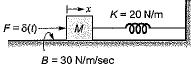The output displacement x(t) for M = 10 kg is given by

Solution:

The differential equation for the given system is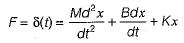or,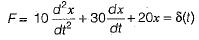Taking Laplace transform on both sides, we get: (10s2 + 30s + 20) X(s) = 1
or,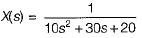or,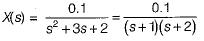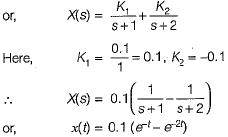QUESTION: 3

### Two wheels are mechanically coupled as shown in figure below.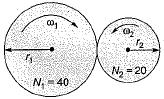Here, ω1 and ω2 = angular velocities (in rad/s) T1 and T2 = Torque (in N-m) r1 and r2 = radius of the wheels (in cm) θ1 and θ2 = angular displacements (in degrees) N1, and N2 = No. of teeths on wheels It is given that ω1 = 10 rad/s, N1 = 40, N2 - 20, T1 = 5 N-m, r1 = 20 cm and θ1 = 20°. Match List-I with List-ll and select the correct answer using the codes given below the lists: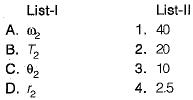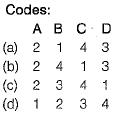Solution:

We know that,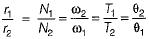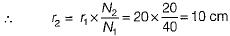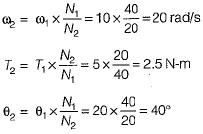QUESTION: 4

The poles-and zeros of the transfer function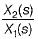for the mechanical system shown below are respectively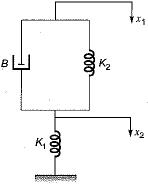Solution:

The differential equation for the given mechanical system is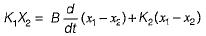Taking Laplace transform on both sides, we get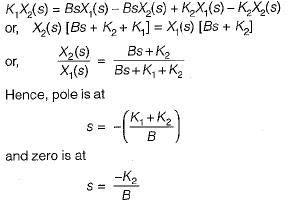QUESTION: 5

Consider the mechanical system shown in figure below.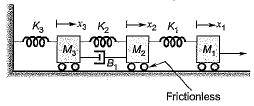Which one of the following circuits shows the force-voltage analogous electrical circuit for the mechanical diagram shown above?

Solution: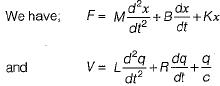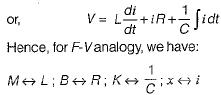Thus, option (d) will be the correct circuit.

QUESTION: 6

Match List-I with List-II and select the correct answer using the codes given below the lists: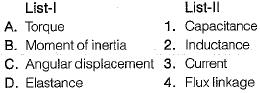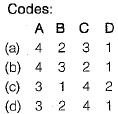Solution:

For mechanical rotational system, we have: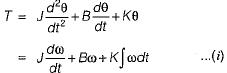For electrical system, we have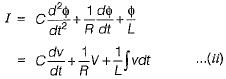Comparing equations (i) and (ii), we have: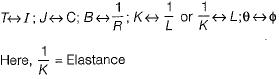QUESTION: 7

Consider the mechanical system shown below: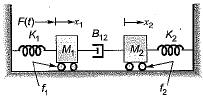The system is described by the differential equations:

Solution:

The free-body diagram for the two masses will be shown as below.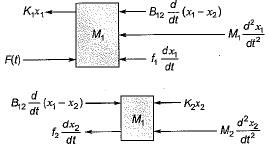Hence, the differential equations for the two masses can be written as follows: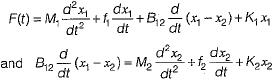QUESTION: 8

Which of the following is not a desirable property of a servomotor in feedback control systems?

Solution:

Relation between electrical control system and rotor speed should be linear.

QUESTION: 9

Consider the following statements related to A.C. servomotors compared to D.C. servomotor used in control system applications.
1. It has less efficiency.
2. It is maintenance free.
3. It gives smooth operation.
4. It gives high power output.

Which of these statements is/are true regarding an A.C. servomotor?

Solution:

An A.C. servomotor doesn’t require brushes compared to a D.C. servomotor. So, it gives maintenance free operation. It’s efficiency is less due to low power output but, gives smooth operation.

QUESTION: 10

A field controlled DC servomotor is a

Solution: